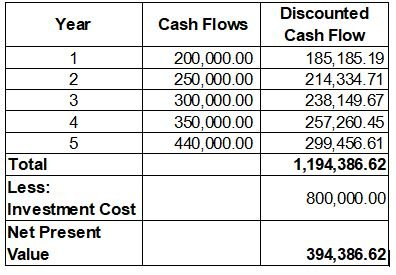# How to do a Discounted Cash Flow Valuation?

299Discounted cash flow (DCF) valuation is one of the Income Approach of the business valuation; the other one is the capitalized earnings approach. DCF valuation is more preferred than the latter since it is more flexible to use, and considers net working capital, leverage, and capital expenditure into the assessment. DCF is computed by discounting the future cash flows using the weighted average cost of capital to derive the net present value (NPV). If the NPV is higher than the total project cost, then the investment is worth it to be considered.

The DCF tells you how much investment you need today to expect the desired return in the future. Discounted cash flow can be used to evaluate a proposed project or the valuation of the business as a whole. Other companies utilized DCF to evaluate their shares or bonds. Even for the purchase of new machinery and equipment, DCF can be a valuable tool to use.

## The Discounted Cash Flow Formula

The discounted cash flow valuation considers the future cash flows, the time value of money, and the discount rate in its computation, which makes it popular among investors. The DCF is computed using the formula below:

DCF = CF1/(1+r)1 + CF2/(1+r)2 + CFn/(1+r)n

where:

• Cash Flow (CF) = The cash flow is the in and out of cash of the business. For a bond, this can be the interest and principal payments.
• r = It is the discount rate that commonly uses the weighted average cost of capital. The discount rate is the expected rate of return for the money invested in the company, which equals the return of other investments with similar risks.
• n = It is the number of years of the projection. It can be a short-term or a long-term forecast. For the purchase of machinery and equipment, short might be enough. But for business valuation as a whole, the long-term projection might be necessary to capture a more accurate result.

## DCF Calculation Example

Let’s say that the expected cash flows for five years are \$200,000; \$250,000; \$300,000; \$350,000; and \$440,000. The weighted average cost of capital is 8%, and the initial investment is \$800,000. The total discounted cash flows amounted to \$1,194,386.62, which means that the net present value is \$394,386.62 after deducting the investment cost. Given that the net present value exceeds the investment cost, the investment is worth it to pursue.## Conclusion

By using the DCF valuation, you can now have a basis that will help you in the decision-making about pursuing your business idea in mind. Since the DCF valuation method considers the time value of money and discount rate, you have a forecast of what you will gain at the end of your investment in today’s value and decide from there if you want to push through your plan. There are widely available financial model templates online that include DCF valuation for the analysis. eFinancialModels offers financial model templates prepared by industry experts to aid you in the preparation of your business valuation.